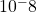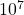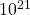### Objective Questions on Basics of Electronics

Page 2

Next Page

Welcome to the second page of Objective questions on basics of electronics-2 to prepare job oriented exams

• The resistance of a material 2 m long and 2 m’ in area of x-section is 16 x 10° Q. Its specific resistance will be 64x 10° Qm

• The SI unit of conductivity is mho/m

• The value of o (temperature co-efficient of resistance) depends upon nature of the material and temperature

• A copper wire of resistance RO is stretched till its length is increased by n times of its original length. Its resistance now will be n°R.

• The resistivity of a material is 2xΩm. What will be the resistance of a hollow pipe of the material of length 1 m and having inner and outer radii 10 cm and 20 cm respectively? 21xQ

• A piece of aluminium (Al) and germanium (Ge) are called from 71 K to T2 K. The resistance of aluminium decreases and that of germanium increases

• At OK, germanium behaves as an insulator

• The example of non-ohmic resistance is carbon resistance

• A copper wire is stretched so that its length is increased by 0.1%. The change in its resistance is 0.2 %

• if an electric current is passed through a nerve, the man BEGINS TO WEEP

• Which one of the following is the best conductor of electricity? Silver
• The temperature co-efficient of resistance of a wire is 000125 per °C. At 300 K, its resistance is 1Ω. The resistance of the wire will be 2Ω at 1400 K

• Ampere – hour is the unit of quantity of electricity

• The resistance of a conductor is 5 Q at 50°C and 6Ω at 100°C. What is its resistance at 0°C?

• Fig. 1.16 shows the temperature / resistance graph of a conductor. The value of α0, is 0.005/°C

• Referring to Fig. 1.16, the value of α50 will be 0-004°C

• Referring to Fig. 1.17, the value of a0, is 1/30 per °C

• Referring to Fig. 1.17, the value of R40, will be 702

• Referring to Fig. 1.17, the value of a, will 1/70 per °C

• The value of a, of a conductor is 1/236 per °C. The value of α18 will be 1/254 per °C

• The value of α25 of a conductor is 1/255 per “C. The value of α20, will be 1/250 per°C

• The value of α50 of a conductor is 1/255 per “C. The value of α0, will be 1/180 per°C

• The number of free electrons passing through the filament of an electric lamp in one hour when the current through the filament is 0-32 A will be 7.2 x• In which of the following substances the resistance decreases with increase in temperature? carbon

• A wire having very high value of conductance is said to be very good conductor

• An electric heater is marked 27000 W, 200V. The resistance of the coil is 20Ω

• The current in a circuit having constant 9 times